This function plots a scatter plot of a term poly.term against a response variable x and adds - depending on the amount of numeric values in poly.degree - multiple polynomial curves. A loess-smoothed line can be added to see which of the polynomial curves fits best to the data.

sjp.poly(
x,
poly.term,
poly.degree,
poly.scale = FALSE,
fun = NULL,
axis.title = NULL,
geom.colors = NULL,
geom.size = 0.8,
show.loess = TRUE,
show.loess.ci = TRUE,
show.p = TRUE,
show.scatter = TRUE,
point.alpha = 0.2,
point.color = "#404040",
loess.color = "#808080"
)

Arguments

x

A vector, representing the response variable of a linear (mixed) model; or a linear (mixed) model as returned by lm or lmer.

poly.term

If x is a vector, poly.term should also be a vector, representing the polynomial term (independent variabl) in the model; if x is a fitted model, poly.term should be the polynomial term's name as character string. See 'Examples'.

poly.degree

Numeric, or numeric vector, indicating the degree of the polynomial. If poly.degree is a numeric vector, multiple polynomial curves for each degree are plotted. See 'Examples'.

poly.scale

Logical, if TRUE, poly.term will be scaled before linear regression is computed. Default is FALSE. Scaling the polynomial term may have an impact on the resulting p-values.

fun

Linear function when modelling polynomial terms. Use fun = "lm" for linear models, or fun = "glm" for generalized linear models. When x is not a vector, but a fitted model object, the function is detected automatically. If x is a vector, fun defaults to "lm".

axis.title

Character vector of length one or two (depending on the plot function and type), used as title(s) for the x and y axis. If not specified, a default labelling is chosen. Note: Some plot types may not support this argument sufficiently. In such cases, use the returned ggplot-object and add axis titles manually with labs. Use axis.title = "" to remove axis titles.

geom.colors

user defined color for geoms. See 'Details' in plot_grpfrq.

geom.size

size resp. width of the geoms (bar width, line thickness or point size, depending on plot type and function). Note that bar and bin widths mostly need smaller values than dot sizes.

show.loess

Logical, if TRUE, an additional loess-smoothed line is plotted.

show.loess.ci

Logical, if TRUE, a confidence region for the loess-smoothed line will be plotted.

show.p

Logical, if TRUE (default), p-values for polynomial terms are printed to the console.

show.scatter

Logical, if TRUE (default), adds a scatter plot of data points to the plot.

point.alpha

Alpha value of point-geoms in the scatter plots. Only applies, if show.scatter = TRUE.

point.color

Color of of point-geoms in the scatter plots. Only applies, if show.scatter = TRUE.

loess.color

Color of the loess-smoothed line. Only applies, if show.loess = TRUE.

A ggplot-object.

Details

For each polynomial degree, a simple linear regression on x (resp. the extracted response, if x is a fitted model) is performed, where only the polynomial term poly.term is included as independent variable. Thus, lm(y ~ x + I(x^2) + ... + I(x^i)) is repeatedly computed for all values in poly.degree, and the predicted values of the reponse are plotted against the raw values of poly.term. If x is a fitted model, other covariates are ignored when finding the best fitting polynomial.

This function evaluates raw polynomials, not orthogonal polynomials. Polynomials are computed using the poly function, with argument raw = TRUE.

To find out which polynomial degree fits best to the data, a loess-smoothed line (in dark grey) can be added (with show.loess = TRUE). The polynomial curves that comes closest to the loess-smoothed line should be the best fit to the data.

Examples

data(efc)
# linear fit. loess-smoothed line indicates a more
# or less cubic curve
sjp.poly(efc$c160age, efc$quol_5, 1)
#> Polynomial degrees: 1
#> ---------------------
#> p(x^1): 0.000
#>
#> geom_smooth() using formula 'y ~ x'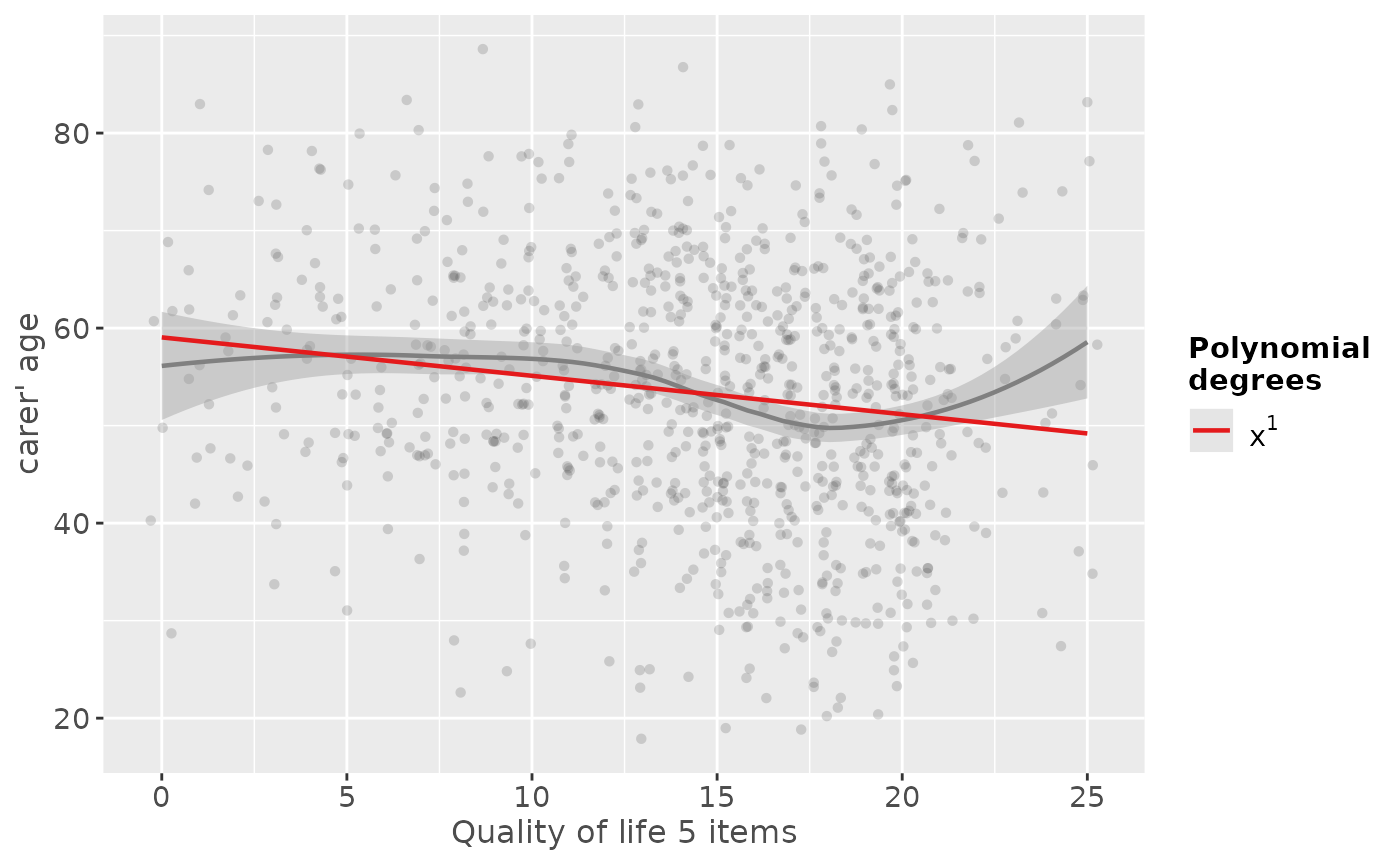sjp.poly(efc$c160age, efc$quol_5, 2)
#> Polynomial degrees: 2
#> ---------------------
#> p(x^1): 0.078
#> p(x^2): 0.533
#>
#> geom_smooth() using formula 'y ~ x'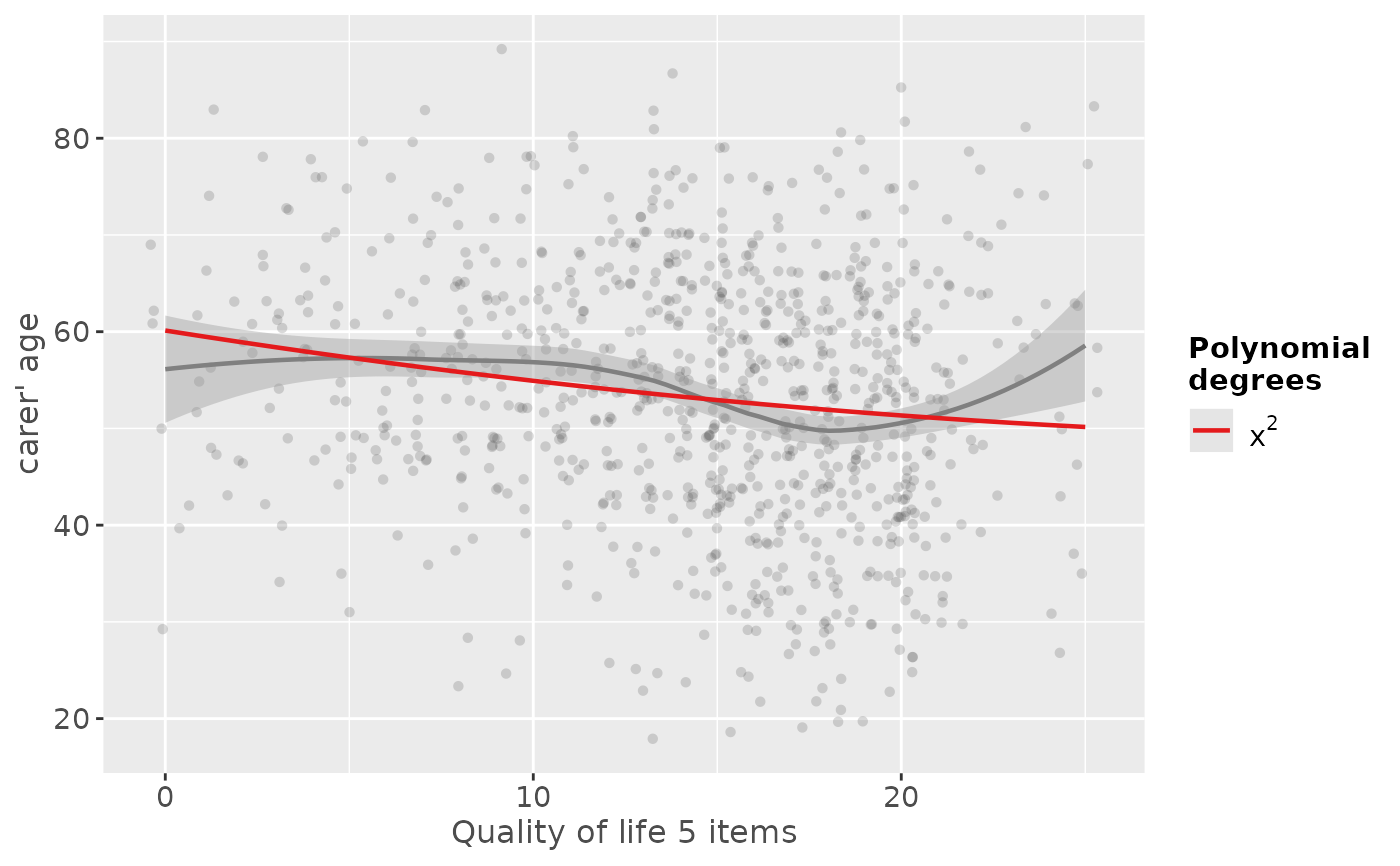# linear to cubic fit
sjp.poly(efc$c160age, efc$quol_5, 1:4, show.scatter = FALSE)
#> Polynomial degrees: 1
#> ---------------------
#> p(x^1): 0.000
#>
#> Polynomial degrees: 2
#> ---------------------
#> p(x^1): 0.078
#> p(x^2): 0.533
#>
#> Polynomial degrees: 3
#> ---------------------
#> p(x^1): 0.012
#> p(x^2): 0.001
#> p(x^3): 0.000
#>
#> Polynomial degrees: 4
#> ---------------------
#> p(x^1): 0.777
#> p(x^2): 0.913
#> p(x^3): 0.505
#> p(x^4): 0.254
#>
#> geom_smooth() using formula 'y ~ x'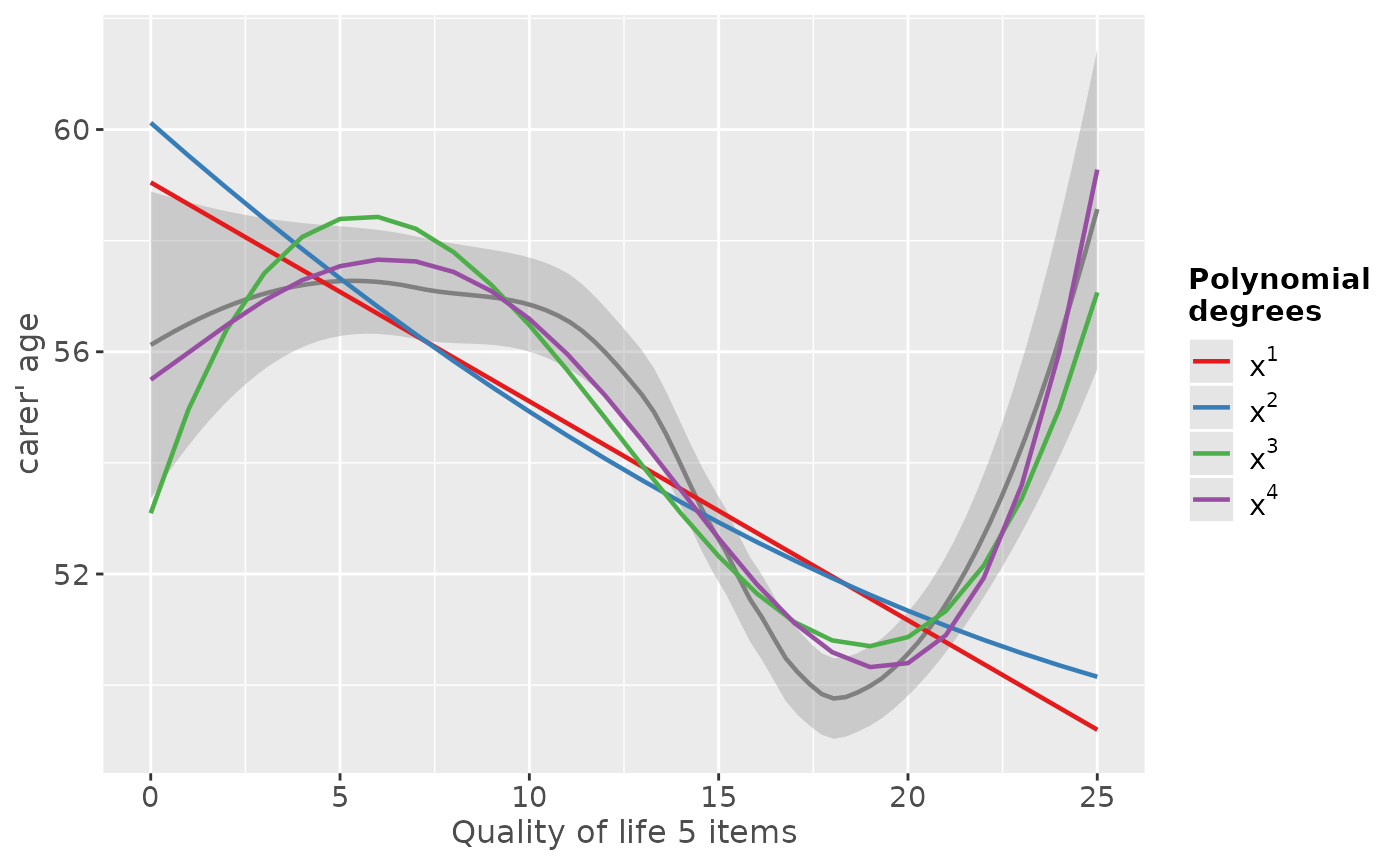# fit sample model
fit <- lm(tot_sc_e ~ c12hour + e17age + e42dep, data = efc)
# inspect relationship between predictors and response
plot_model(fit, type = "slope")
#> geom_smooth() using formula 'y ~ x'
#> geom_smooth() using formula 'y ~ x'
#> Warning: pseudoinverse used at 4.015
#> Warning: reciprocal condition number  2.8666e-15
#> Warning: There are other near singularities as well. 1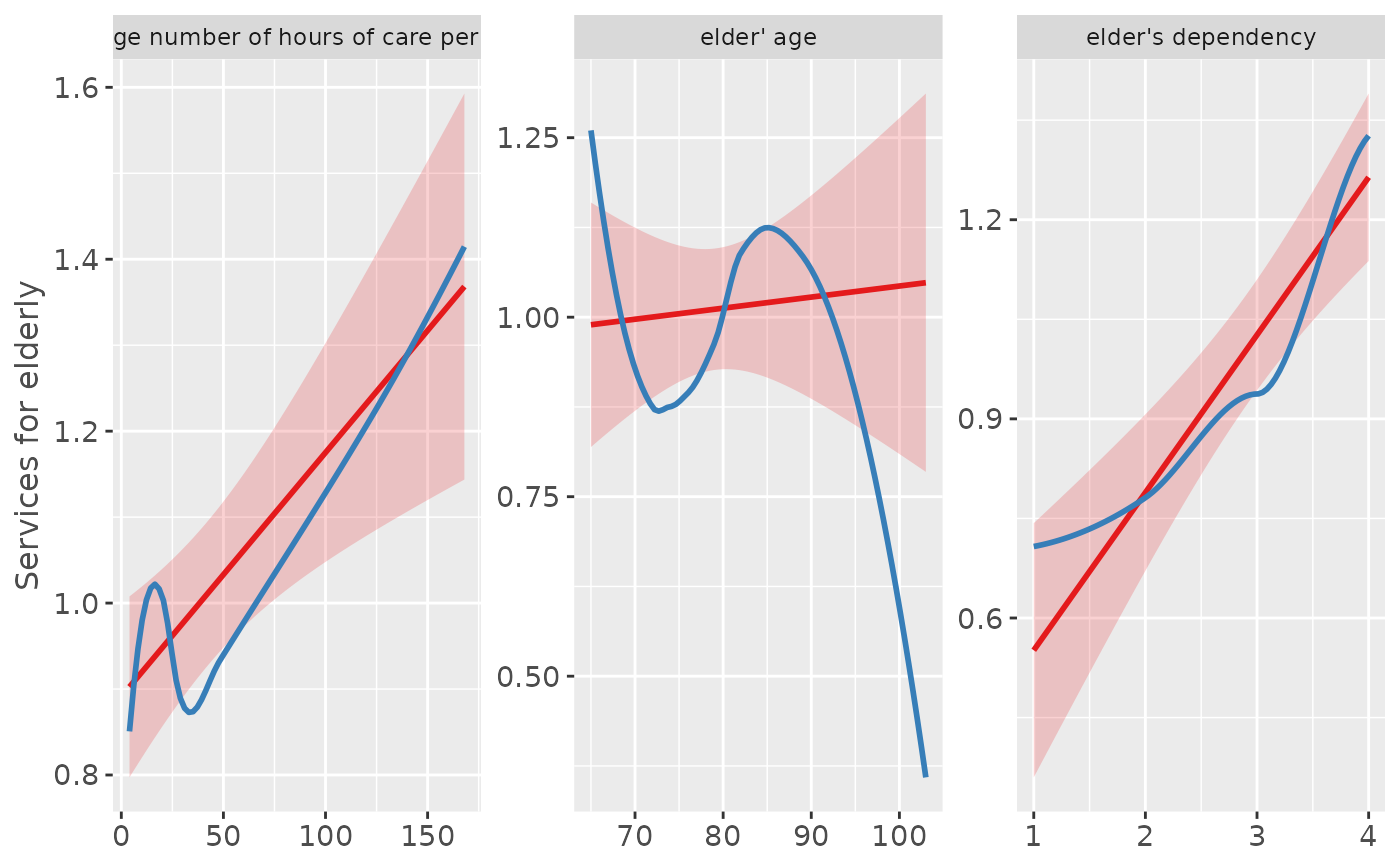# "e17age" does not seem to be linear correlated to response
# try to find appropiate polynomial. Grey line (loess smoothed)
# indicates best fit. Looks like x^4 has the best fit,
# however, only x^3 has significant p-values.
sjp.poly(fit, "e17age", 2:4, show.scatter = FALSE)
#> Polynomial degrees: 2
#> ---------------------
#> p(x^1): 0.734
#> p(x^2): 0.721
#>
#> Polynomial degrees: 3
#> ---------------------
#> p(x^1): 0.010
#> p(x^2): 0.011
#> p(x^3): 0.011
#>
#> Polynomial degrees: 4
#> ---------------------
#> p(x^1): 0.234
#> p(x^2): 0.267
#> p(x^3): 0.303
#> p(x^4): 0.343
#>
#> geom_smooth() using formula 'y ~ x'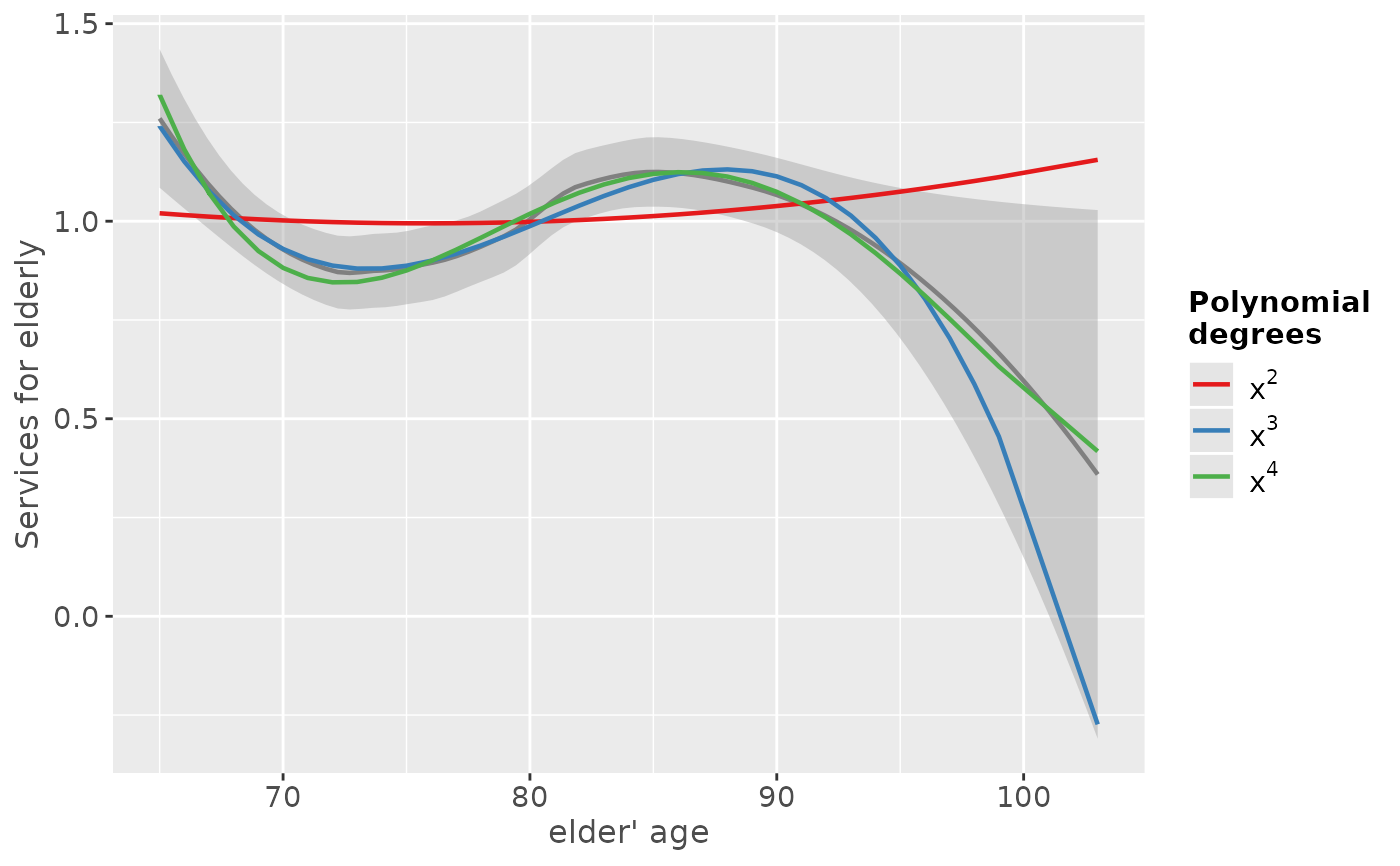if (FALSE) {
# fit new model
fit <- lm(tot_sc_e ~ c12hour + e42dep + e17age + I(e17age^2) + I(e17age^3),
data = efc)
# plot marginal effects of polynomial term
plot_model(fit, type = "pred", terms = "e17age")}